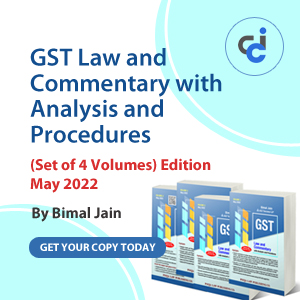# Demand & Time Liability of Savings Bank AccountGuest , 09 August 2012

Demand and time liability of savings deposits

Demand liability is one which is demandable at any point of time whereas time liability is one which is core portion and not demandable for a longer period. when interest on savings deposits was paid on month minimum balance (from 11 to 31 date of the month) the average of minimum balance could be reckoned from interest formulae as under.

Monthly interest = Principal * Rate / 1200

From that minimum balance was derived as

Principal = Half yearly interest paid / Rate *1200 / 6

This principal was divided by sum of average balance for 6 months which is a core portion or time liability and the rest is demand liability.

This formulae was not proper because in that case only volatility between 11th to 31st days was counted and volatility of first 10 days was ignored as interest was paid on the minimum balance of 11 to 31st dates. So, the minimum balance predicted from the interest formulae was not giving the actual core portion. (e.g. if the opening balance of the month is rs.10000/-, and if Rs  5000/- credited on 9th date than minimum balance as per this formulae will be Rs15000/- as interest will be given on Rs 15000/- but in actual case the right minimum balance is Rs 10000/- only.) So as per old formulae if there is a single transaction of credit before 11th date, the demand portion is less than actual i.e. core portion is wrongly more than actual Rs 15000/- instead of Rs 10000/-. Further in the new era of differential interest rate, one can’t use this interest based formulae to get minimum balance.

In my opinion the core/demandable portion has nothing to do with interest formulae. But to get minimum balance from the old ledgers was cumbersome and tedious as well as lengthy process. To simplify this, the minimum balance was predicted from interest formulae as interest was paid on minimum balance and average balance could be easily found from general balance ledgers. So, the interest formula was used to get minimum balance.

To simplify, considering the formulae the actual concept is of minimum & average balance only.

(Demand = withdrawal and rest minimum balance is core or time portion)

In the current situation when interest is paid on daily product the minimum balance cannot be derived from the earlier used formulae. in that case we have to derive minimum as well as average balance from the data’s directly and demand liabilities of savings can be derived from the formulae given below.

Demandable savings portion (%) = 100 - (average of last 6 months minimum balance / average of last 6 months average balance *100)

e.g.

 Month Minimum balance Average balance % of Time liability % of Demand liability April 12500 14500 86 14 May 13000 15000 87 13 June 14000 16000 88 13 July 13500 15500 87 13 August 13800 15600 88 12 Sep11 14000 15900 88 12 Sum of 6 months 80800 92500 87 13 Average of 6 months 13467 15417 87 13

If we want we can also calculate this at quarterly or yearly interval by taking respective average balances instead of half yearly interval. As in earlier days interest was paid at half yearly interval, this calculation also done for the same period to get minimum balance from interest paid amount. But now we are doing quarterly closing we can introduce quarterly calculation also. In the aggressive marketing and differential interest rate era, we should consider average of last 3 months instead of half yearly interval.

By: Dipesh S.Shah

Member (Account Deleted)
(.)
Category Accounts   Report

2 Likes   107 Shares   46110 Views

### Popular ArticlesCCI Articles